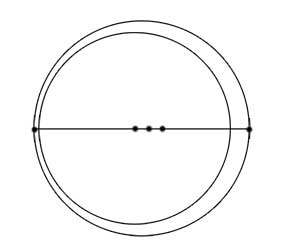SEARCH HOMEMath Central Quandaries & QueriesGina, a student: A satellite has an elliptical orbit around the earth with one focus at the earth’s center, E. The earth’s radius is 4,000 miles. The highest point that the satellite is from the surface of the earth is 800 miles, and the lowest is 200 miles. What is the eccentricity of the satellite’s orbit?Hi Gina,

For my diagram, I set one focus at the centre of the Earth so that means that the focus is 4200 miles from the satellite at its lowest point and 4800 miles from the satellite at its highest point. The lowest and highest points of the satellite will be the vertices of the major axis, where the major axis=2a where a is the distance from a vertex to the centre. This means we know that the major axis is 9000 miles.The eccentricity is ε=c/a where c is the distance from the centre to a focus. What is the value of c? What is the eccentricity?

Hope this helps,

JaniceMath Central is supported by the University of Regina and The Pacific Institute for the Mathematical Sciences.